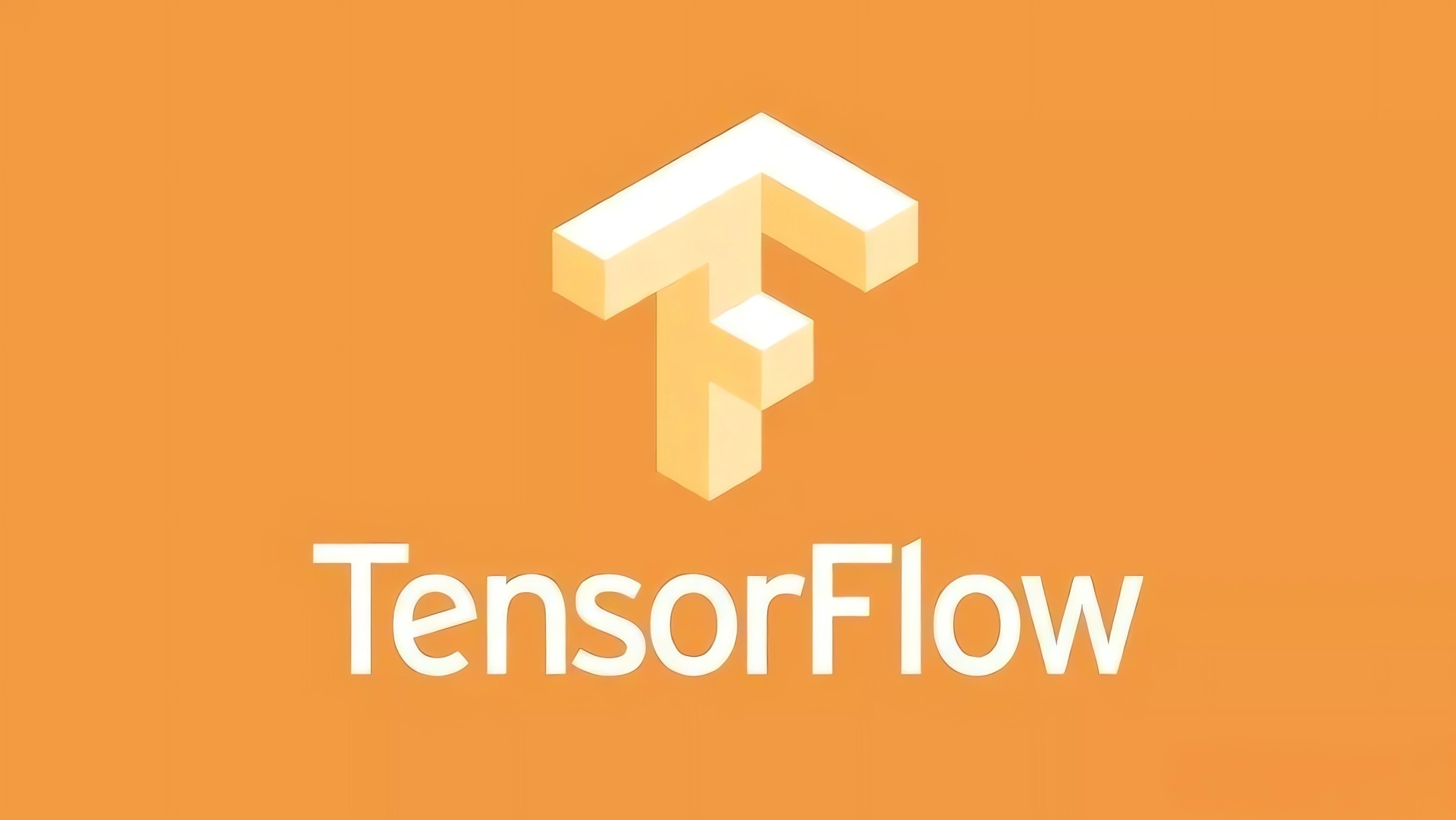``````import tensorflow as tf

def model_fn(features, labels, mode, params):
predictions = ...
loss = ...
train_op = ...
metric_ops = ...
return tf.estimator.EstimatorSpec(
mode=mode,
predictions=predictions,
loss=loss,
train_op=train_op,
eval_metric_ops=metric_ops)

params = ...
run_config = tf.contrib.learn.RunConfig(model_dir=FLAGS.output_dir)
estimator = tf.estimator.Estimator(
model_fn=model_fn, config=run_config, params=params)
``````

``````def input_fn():
features = ...
labels = ...
return features, labels

estimator.train(input_fn=input_fn, max_steps=...)
``````

``````estimator.evaluate(input_fn=input_fn)
``````

``````experiment = tf.contrib.learn.Experiment(
estimator=estimator,
train_input_fn=train_input_fn,
eval_input_fn=eval_input_fn)
``````

``````experiment.train_and_evaluate()
``````

``````import tensorflow as tf

tf.flags.DEFINE_string("output_dir", "", "Optional output dir.")
tf.flags.DEFINE_string("schedule", "train_and_evaluate", "Schedule.")
tf.flags.DEFINE_string("hparams", "", "Hyper parameters.")

FLAGS = tf.flags.FLAGS

def experiment_fn(run_config, hparams):
estimator = tf.estimator.Estimator(
model_fn=make_model_fn(),
config=run_config,
params=hparams)
return tf.contrib.learn.Experiment(
estimator=estimator,
train_input_fn=make_input_fn(tf.estimator.ModeKeys.TRAIN, hparams),
eval_input_fn=make_input_fn(tf.estimator.ModeKeys.EVAL, hparams))

def main(unused_argv):
run_config = tf.contrib.learn.RunConfig(model_dir=FLAGS.output_dir)
hparams = tf.contrib.training.HParams()
hparams.parse(FLAGS.hparams)

estimator = tf.contrib.learn.learn_runner.run(
experiment_fn=experiment_fn,
run_config=run_config,
schedule=FLAGS.schedule,
hparams=hparams)

if __name__ == "__main__":
tf.app.run()
``````

``````def input_fn():
features = ...
labels = ...
return features, labels
``````